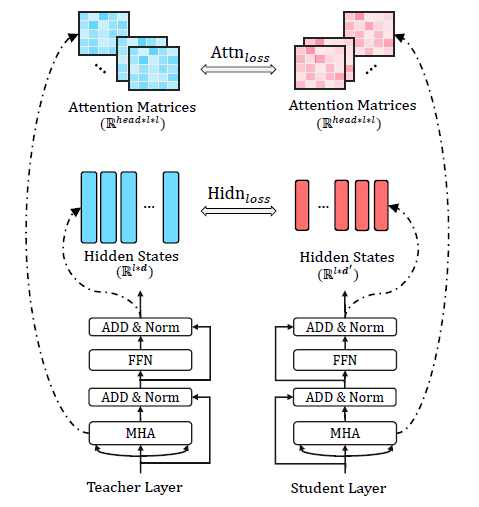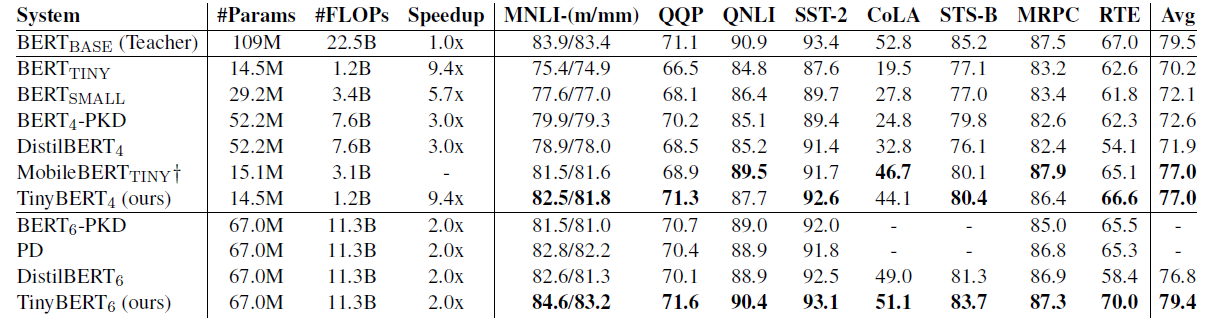# Review — TinyBERT: Distilling BERT for Natural Language Understanding

## TinyBERT, Outperforms MobileBERT, Much Smaller Than BERT

TinyBERT: Distilling BERT for Natural Language Understanding
TinyBERT, by Huazhong University of Science and Technology, Huawei Noah’s Ark Lab, and Huawei Technologies Co., Ltd.
2020 EMNLP, Over 600 Citations (Sik-Ho Tsang @ Medium)
Natural Language Processing, NLP, Language Model, BERT, Transformer, Distillation

• A new two-stage learning framework is proposed for TinyBERT, which performs Transformer Distillation at both the pretraining and task-specific learning stages.

# Outline

1. Preliminaries
2. TinyBERT Knowledge Distillation Losses
3. TinyBERT Knowledge Distillation Stages
4. Experimental Results

# 1. Preliminaries

## 1.1. Transformer Layer

• A standard Transformer layer includes two main sub-layers: multi-head attention (MHA) and fully connected feed-forward network (FFN).
• For MHA, there are three components of queries, keys and values, denoted as matrices Q, K and V respectively. The attention function can be formulated as follows:
• Multi-head attention is defined by concatenating the attention heads from different representation subspaces as follows:
• FFN contains two linear transformations and one ReLU activation:

## 1.2. Knowledge Distillation (KD)

• Knowledge Distillation (KD) aims to transfer the knowledge of a large teacher network T to a small student network S.
• The student network is trained to mimic the behaviors of teacher networks. fT and fS denote the behavior functions of teacher and student networks, respectively. KD can be modeled as minimizing the following objective function:
• where x is the text input and X denotes the training dataset.

# 2. TinyBERT Knowledge Distillation Losses

## 2.1. Problem Formulation

M out of N layers from the teacher model are chosen for the Transformer-layer distillation.

m-th layer of student model learns the information from the g(m)-th layer of teacher model.

• Formally, the student can acquire knowledge from the teacher by minimizing the following objective:
• where Llayer refers to the loss function of a given model layer (e.g., Transformer layer or embedding layer), fm(x) denotes the behavior function induced from the m-th layers and λm is the hyperparameter that represents the importance of the m-th layer’s distillation.

## 2.2. Transformer-Layer DistillationTransformer-layer distillation consists of Attnloss (attention based distillation) and Hidnloss (hidden states based distillation)

## 2.2.1. Attention Based Distillation

• Attention weights learned by BERT can capture rich linguistic knowledge, which includes the syntax and coreference information. It is essential for natural language understanding. MSE is used for the attention based distillation so that the linguistic knowledge can be transferred from teacher (BERT) to student (TinyBERT):
• where h is the number of attention heads.

## 2.2.2. Hidden States Based Distillation

• The knowledge from the output of Transformer layer is also distilled, and the objective is to minimize the hidden states using MSE as follows:
• Since d’ is often smaller than d where d and d’ which are the hidden sizes of teacher and student models respectively, the matrix Wh is a learnable linear transformation, which transforms the hidden states of student network into the same space as the teacher network’s states.

## 2.3. Embedding-Layer Distillation

• where the matrices ES and ET refer to the embeddings of student and teacher networks, respectively. The matrix We is a linear transformation playing a similar role as Wh.

## 2.4. Prediction-Layer Distillation

• Knowledge distillation is used to fit the predictions of teacher model as in distillation. Specifically, the soft cross-entropy loss is used between the student network’s logits against the teacher’s logits:
• where zS and zT are the logits vectors predicted by the student and teacher respectively, CE means the cross entropy loss, and t means the temperature value. t=1 in this paper.

## 2.5. Overall

• To unify, the distillation loss of the corresponding layers between the teacher and the student network is:

# 3. TinyBERT Knowledge DistillationStages

• The application of BERT usually consists of two learning stages: the pre-training and fine-tuning, including the general distillation and the task-specific distillation.

## 3.1. General Distillation

• General distillation helps TinyBERT learn the rich knowledge embedded in pre-trained BERT.
• The original BERT without fine-tuning is used as the teacher and a large-scale text corpus is used as the training data. The Transformer distillation but without prediction-layer distillation, is performed.

However, due to the significant reductions of the hidden/embedding size and the layer number, general TinyBERT performs generally worse than BERT.

• The task-specific distillation further teaches TinyBERT the knowledge from the fine-tuned BERT. The proposed Transformer distillation is re-performed on an augmented task-specific dataset.
• Specifically, the fine-tuned BERT is used as the teacher and a data augmentation method is proposed to expand the task-specific training set.
• Training with more task-related examples, the generalization ability of the student model can be further improved.

## 3.3. Data Augmentation

• Pre-trained language model BERT and GloVe word embeddings are combined to do word-level replacement for data augmentation.
• Specifically, the language model is used to predict word replacements for single-piece words, and the word embeddings are used to retrieve the most similar words as word replacements for multiple-pieces words.
• pt=0.4, Na=20, K=15 are used in the above algorithm.

# 4. Experimental Results

## 4.1. TinyBERT Variants

• TinyBERT4: A tiny student model (the number of layers M=4, the hidden size d’=312, the feedforward/filter size di=1200 and the head number h=12) that has a total of 14.5M parameters.
• BERTBASE (N=12, d=768, di=3072 and h=12) is used as the teacher model that contains 109M parameters.
• TinyBERT6 (M=6, d’=768, di=3072 and h=12) with the same architecture as BERT6-PKD (Sun et al., 2019) and DistilBERT6.

## 4.2. Results on GLUEResults are evaluated on the test set of GLUE official benchmark
• There is a large performance gap between BERTTINY (or BERTSMALL) and BERTBASE due to the dramatic reduction in model size.
• TinyBERT4 is consistently better than BERTTINY on all the GLUE tasks and obtains a large improvement of 6.8% on average.
• TinyBERT4 significantly outperforms the 4-layer state-of-the-art KD baselines (i.e., BERT4-PKD and DistilBERT4) by a margin of at least 4.4%, with 28% parameters and 3.1× inference speedup.
• Compared with the teacher BERTBASE, TinyBERT4 is 7.5× smaller and 9.4× faster in the model efficiency, while maintaining competitive performances.
• TinyBERT is also compared with the 24-layer MobileBERTTINY, which is distilled from 24-layer IB-BERTLARGE. The results show that TinyBERT4 achieves the same average score as the 24-layer model with only 38.7% FLOPs.
• When we the capacity of the model is increased to TinyBERT6, its performance can be further elevated and outperforms the baselines of the same architecture by a margin of 2.6% on average and achieves comparable results with the teacher.

## 4.3. Effects of Learning Procedure

The results indicates that all of the three procedures are crucial for the proposed method.

## 4.4. Effects of Distillation Objective

It is shown that all the proposed distillation objectives are useful. The performance w/o Trm drops significantly from 75.6 to 56.3.

## 4.5. Effects of Mapping Function

• n=g(m): The effects of different mapping functions
• The original TinyBERT uses the uniform strategy, and two typical baselines including top-strategy (g(m)=m+N-M; 0<mM) and bottom-strategy (g(m)=m; 0<mM), are compared.

The uniform strategy covers the knowledge from bottom to top layers of BERTBASE, and it achieves better performances.

• (There are also other results in the appendix, please feel free to read the paper directly.)

## Reference

[2020 EMNLP] [TinyBERT]
TinyBERT: Distilling BERT for Natural Language Understanding

## Language Model

2007 … 2019 [T64] [Transformer-XL] [BERT] [RoBERTa] [GPT-2] [DistilBERT] [MT-DNN] [Sparse Transformer] [SuperGLUE] [FAIRSEQ] 2020 [ALBERT] [GPT-3] [T5] [Pre-LN Transformer] [MobileBERT] [TinyBERT]

--

--